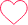# Wipro NTH Coding Question-1

### 4 comments on “Wipro NTH Coding Question-1”

•Chandu

Can I get code in Python please0
•Sweety

how can i get code for this in python6
•Pathan

l=list(map(int,input().split(” “)))
a=int(input())
l1=*len(l)
for i in range(0,a):
for j in range(0,len(l)):
if j==0:
a=0
b=l
elif (j==len(l)-1):
b=0
a=l[len(l)-2]
else:
a=l[j-1]
b=l[j+1]
if (a==b):
l1[j]=0
else:
l1[j]=1
l=l1
l1=*len(l)
for i in range(0,len(l)):
print(l[i],end=” “)
#Hope it might help0
•karthiksaik2000

s = [1,1,1,0,1,1,1,1] # you can also use – input()
op= [0,0,0,0,0,0,0,0]
n = int(input()) # number of test cases
for i in range(n):
for j in range(1,len(s)-1):
op[j]=s[j-1]^s[j+1]
op=0^s
op[-1]=s^0
s = op
op = [0,0,0,0,0,0,0,0]
print(s)0